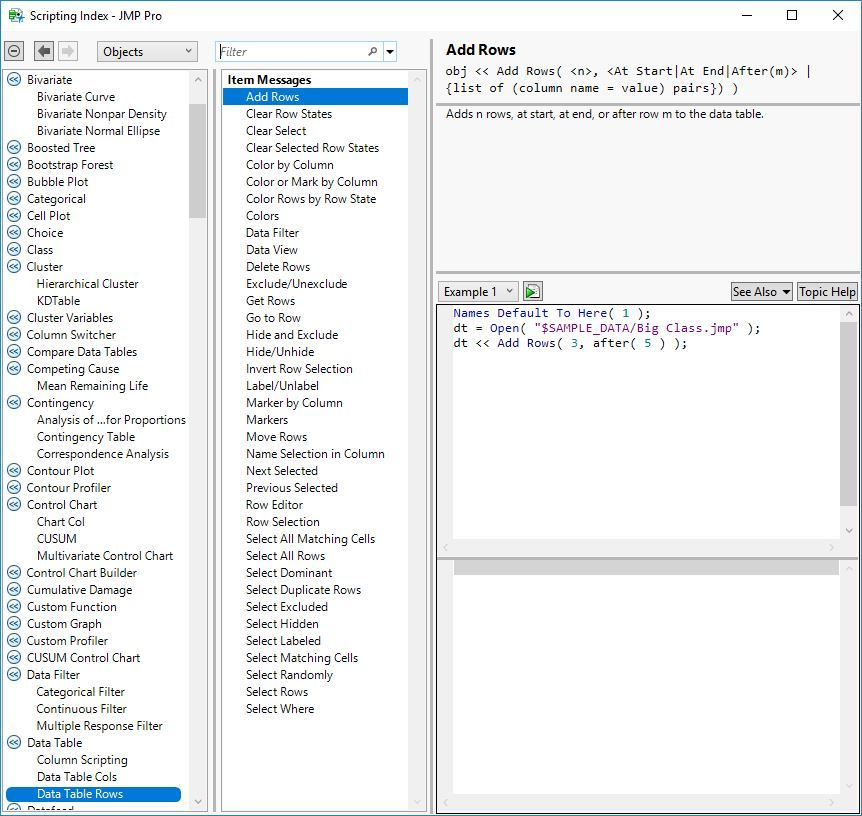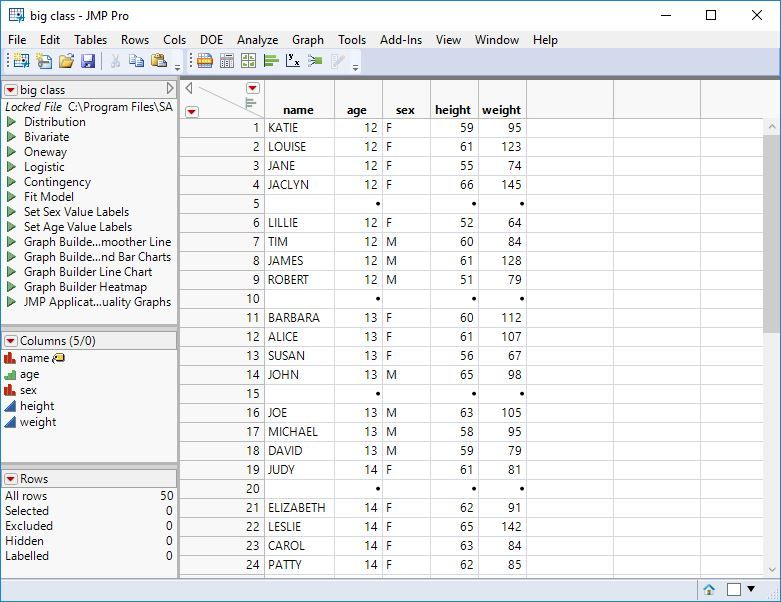Choose Language Hide Translation Bar
Highlighted

## Add rows every x rows

Dear community, i've got a huge table and would like to add one row every 4 rows (4 rows data, adding 1 row, moving the rest of the data downwards, then adding 1 row after 4 rows of data aso).

I have no clue how to achieve that with a script. Can anyone kindly help me with this topic?

7 REPLIES 7
Highlighted

## Re: Add rows every x rows

See Help > Scripting Index:The << Add Rows message to the data table will do what you want. The optional After argument identifies the location, so you can space the insertions every 4 rows.

Use the For() function to iterate over the data table. The iteration will be easiest if you start at the end and insert on the way to the top. The insertions will each length the data table and complicate the counting if you start at the top.

Learn it once, use it forever!
Highlighted

## Re: Add rows every x rows

Just to add to @markbailey suggestion, here is a real simple example

``````names default to here(1);
dt=open("\$SAMPLE_DATA/big class.jmp");

For(i=N Rows(dt),i>=1,i=i-4,
);``````
Jim
Highlighted

## Re: Add rows every x rows

When I run the code, I get the following error:

```Name Unresolved: after in access or evaluation of 'after' , after( i ) /*###*/argument is wrong type in access or evaluation of 'after' , after( i ) /*###*/
In the following script, error marked by /*###*/
Names Default To Here( 1 );
dt = Open( "\$SAMPLE_DATA/big class.jmp" );
For( i = N Rows( dt ), i >= 1, i = i - 4,
dt << add rows( 1, after( i ) /*###*/ )
);```

Highlighted

## Re: Add rows every x rows

It works for me:``````Names Default To Here( 1 );
dt = Open( "\$SAMPLE_DATA/big class.jmp" );
For( i = N Rows( dt ), i >= 1, i = i - 4,
dt << Add Rows( 1, After( i ) )
);``````
Learn it once, use it forever!
Highlighted

## Re: Add rows every x rows

I am running JMP13 but I don't think that is the cause of the issue.  Think I will contact tech support.

Highlighted

## Re: Add rows every x rows

I bet you are using JMP 13 or earlier.  The "After" capability was added in JMP 14.  Here is alternative way to do what you need

``````names default to here(1);
dt=open("\$SAMPLE_DATA/big class.jmp");

dt4 = New Table("Results");

For(i=1,i<=n rows(dt), i=i+3,
dt<<select where(Row()>=i & Row()<=i+3);
dtTemp = dt << subset(selected rows(1), selected cols(0));
dt4 << concatenate(dtTemp, append to first table);
close( dtTemp, nosave );
);``````

Jim
Highlighted

## Re: Add rows every x rows

That did the trick.  Thanx.

Article Labels